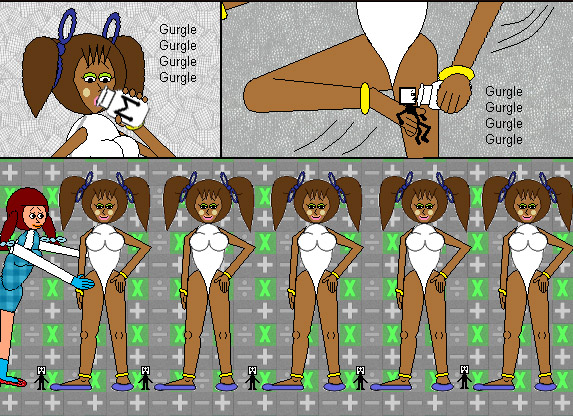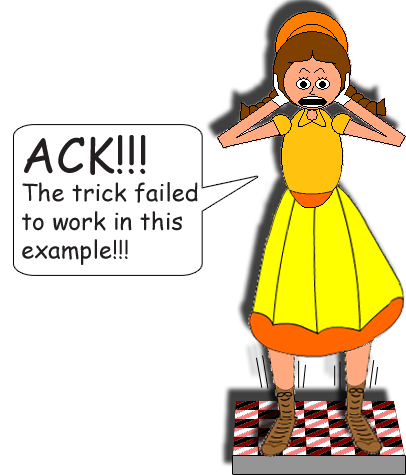# Digit Cloning With Multiplication!

## Surely, you know what happens when you multiply single-digit numbers by 11, right?Mr. Eleven Primen, Distant Member of the Digit Family

12 × 101 = 1,212

25 × 101 = 2,525

36 × 101 = 3,636

47 × 101 = 4,747

59 × 101 = 5,959

## If you multiply 3-digit numbers by 1,001...

123 × 1,001 = 123,123

279 × 1,001 = 279,279

380 × 1,001 = 380,380

456 × 1,001 = 456,456

517 × 1,001 = 517,517

## If you multiply 4-digit numbers by 10,001...

1,849 × 10,001 = 18,491,849

2,084 × 10,001 = 20,842,084

3692 × 10,001 = 36,923,692## For 7-digit numbers, multiply by 10,000,001.

The more digits the multiplicand has, the more zeroes you must put in the "number sandwich". (Consider the 1's the bread slices) The number sandwich, as I called it, is the multiplier. By multiplying correctly, you get these special products which make it look like you cloned all the digits in the multiplicand! But how many zeroes should you put between the 1's? Simple! [The number of digits the multiplicand has] - 1 = [The number of zeroes you should put in the multiplying number sandwich!]

## Here's the formula again below with variables:

x - 1 = y

x = The number of digits the multiplicand has

y = The number of zeroes you should put in the multiplying number sandwich!

## But also, consider this function:

10x + 1 = y

The variable y IS the number sandwich in this function. All of the number sandwiches above are equal to 1 + a power of 10.If you multiply by the correct number sandwich, you'll clone all of the multiplicand's digits, which you'll get in the product! The digits are cloned just like how Pixelman & Lady Fingersandtoes cloned themselves by drinking Multi-Milk in the cartoon above!

But beware, because if the number sandwich doesn't have enough zeroes, something like this will happen:

144 × 101 = 14,544If it has too many zeroes, something like this will happen:

144 × 100,001 = 14,400,144

## But guess what...You can use double-decker number sadwiches, triple-deckers, quadruple-deckers, et cetera, which makes this math trick even cooler!

54 × 10,101 = 545,454

216 × 1,001,001,001 = 216,216,216,216

78 × 101,010,101 = 7,878,787,878

## But put the exact same amount of zeroes between the 1's! (The 1's are the bread slices of the number sandwiches!) Finally, use 1's & zeroes only; don't use any of the other 8 digits in the number sandwiches or this math trick just won't work!

Something like this will happen:

27 × 484 = 13,068